# 比特币白皮书：一种点对点的电子现金系统#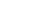## 3. 时间戳服务器(Timestamp server)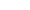## 4. 工作量证明（Proof-of-Work）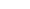## 5. 网络

• 1) 新的交易向全网进行广播；
• 2) 每一个节点都将收到的交易信息纳入一个区块中；
• 3) 每个节点都尝试在自己的区块中找到一个具有足够难度的工作量证明；
• 4) 当一个节点找到了一个工作量证明，它就向全网进行广播；
• 5) 当且仅当包含在该区块中的所有交易都是有效的且之前未存在过的，其他节点才认同该区块的有效性；
• 6) 其他节点表示他们接受该区块，而表示接受的方法，则是在跟随该区块的末尾，制造新的区块以延长该链条，而将被接受区块的随机散列值视为先于新区快的随机散列值。

## 7. 回收硬盘空间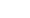## 8. 简化的支付确认（Simplified Payment Verification）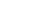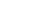## 10. 隐私（Privacy）## 11. 计算```#include double AttackerSuccessProbability(double q, int z) { double p = 1.0 - q; double lambda = z * (q / p); double sum = 1.0; int i, k; for (k = 0; k <= z; k++) { double poisson = exp(-lambda); for (i = 1; i <= k; i++) poisson *= lambda / i; sum -= poisson * (1 - pow(q / p, z - k)); } return sum; }```

z=0 P=1.0000000
z=1 P=0.2045873
z=2 P=0.0509779
z=3 P=0.0131722
z=4 P=0.0034552
z=5 P=0.0009137
z=6 P=0.0002428
z=7 P=0.0000647
z=8 P=0.0000173
z=9 P=0.0000046
z=10 P=0.0000012

z=0 P=1.0000000
z=5 P=0.1773523
z=10 P=0.0416605
z=15 P=0.0101008
z=20 P=0.0024804
z=25 P=0.0006132
z=30 P=0.0001522
z=35 P=0.0000379
z=40 P=0.0000095
z=45 P=0.0000024
z=50 P=0.0000006

q=0.10 z=5
q=0.15 z=8
q=0.20 z=11
q=0.25 z=15
q=0.30 z=24
q=0.35 z=41
q=0.40 z=89
q=0.45 z=340

## 12.结论

1. W Dai（戴伟）,a scheme for a group of untraceable digital pseudonyms to pay each other with money and to enforce contracts amongst themselves without outside help（一种能够借助电子假名在群体内部相互支付并迫使个体遵守规则且不需要外界协助的电子现金机制）, “B-money”, http://www.weidai.com/bmoney.txt, 1998
2. H. Massias, X.S. Avila, and J.-J. Quisquater, “Design of a secure timestamping service with minimal trust requirements,”（在最小化信任的基础上设计一种时间戳服务器） In 20th Symposium on Information Theory in the Benelux, May 1999.
3. S. Haber, W.S. Stornetta, “How to time-stamp a digital document,” （怎样为电子文件添加时间戳）In Journal of Cryptology, vol 3, No.2, pages 99-111, 1991.
4. D. Bayer, S. Haber, W.S. Stornetta, “Improving the efficiency and reliability of digital time-stamping,”（提升电子时间戳的效率和可靠性） In Sequences II: Methods in Communication, Security and Computer Science, pages 329-334, 1993.
5. S. Haber, W.S. Stornetta, “Secure names for bit-strings,”（比特字串的安全命名） In Proceedings of the 4th ACM Conference on Computer and Communications Security, pages 28-35, April 1997. on Computer and Communications Security, pages 28-35, April 1997.
6. A. Back, “Hashcash – a denial of service counter-measure,”（哈希现金——拒绝服务式攻击的克制方法）http://www.hashcash.org/papers/hashcash.pdf, 2002.
7. R.C. Merkle, “Protocols for public key cryptosystems,” （公钥密码系统的协议）In Proc. 1980 Symposium on Security and Privacy, IEEE Computer Society, pages 122-133, April 1980.
S. Haber, W.S. Stornetta, “Secure names for bit-strings,”（比特字串安全命名） In Proceedings of the 4th ACM Conference on Computer and Communications Security, pages 28-35, April 1997. on Computer and Communications Security, pages 28-35, April 1997.
H. Massias, X.S. Avila, and J.-J. Quisquater, “Design of a secure timestamping service with minimal trust requirements,”（在最小化信任的条件下设计一种时间戳服务器） In 20th Symposium on Information Theory in the Benelux, May 1999.
8. W. Feller, “An introduction to probability theory and its applications,” （概率学理论与应用导论）1957
Tagged

### 说点什么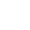Top